MathScore EduFighter is one of the best math games on the Internet today. You can start playing for free!

## Compare Rectangle Area and Perimeter - Sample Math Practice Problems

The math problems below can be generated by MathScore.com, a math practice program for schools and individual families. References to complexity and mode refer to the overall difficulty of the problems as they appear in the main program. In the main program, all problems are automatically graded and the difficulty adapts dynamically based on performance. Answers to these sample questions appear at the bottom of the page. This page does not grade your responses.

See some of our other supported math practice problems.

### Complexity=2, Mode=1

Find the area. Answer with proper units. To represent m 2, use "sq m". Sample answer:"5 sq m".

 1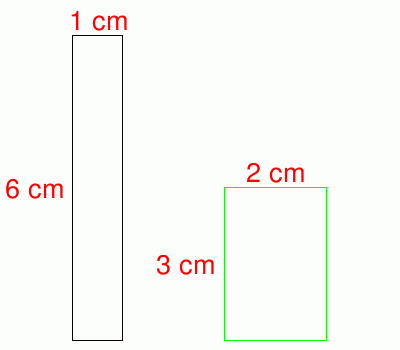Area of either Rectangle= Perimeter of Black Rectangle= Perimeter of Green Rectangle= 2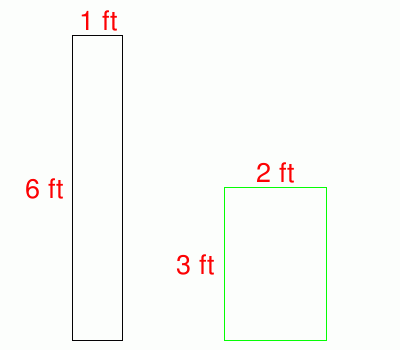Area of either Rectangle= Perimeter of Black Rectangle= Perimeter of Green Rectangle=

### Complexity=4, Mode=1

Find the area. Answer with proper units. To represent m 2, use "sq m". Sample answer:"5 sq m".

 1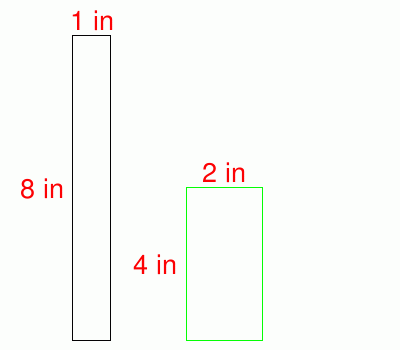Area of either Rectangle= Perimeter of Black Rectangle= Perimeter of Green Rectangle= 2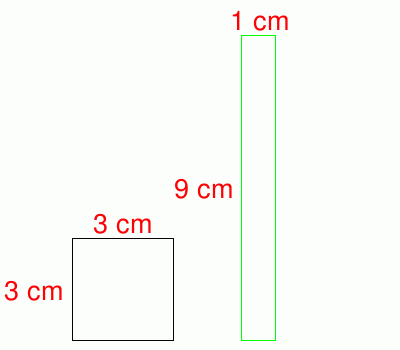Area of either Rectangle= Perimeter of Black Rectangle= Perimeter of Green Rectangle=

### Complexity=6, Mode=1

Find the area. Answer with proper units. To represent m 2, use "sq m". Sample answer:"5 sq m".

 1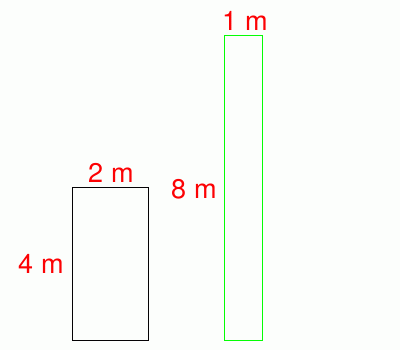Area of either Rectangle= Perimeter of Black Rectangle= Perimeter of Green Rectangle= 2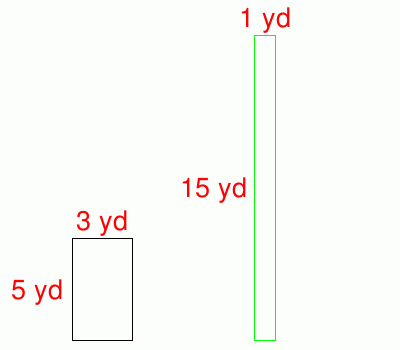Area of either Rectangle= Perimeter of Black Rectangle= Perimeter of Green Rectangle=

### Complexity=8, Mode=1

Find the area. Answer with proper units. To represent m 2, use "sq m". Sample answer:"5 sq m".

 1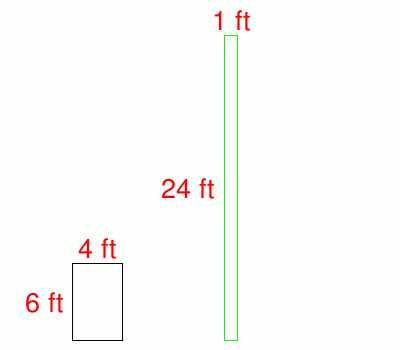Area of either Rectangle= Perimeter of Black Rectangle= Perimeter of Green Rectangle= 2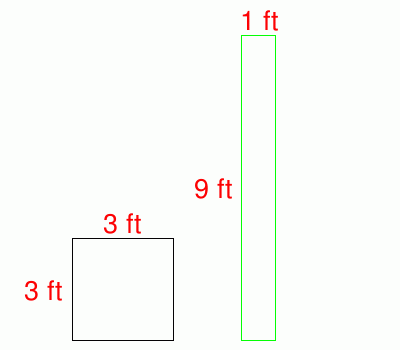Area of either Rectangle= Perimeter of Black Rectangle= Perimeter of Green Rectangle=

### Complexity=2, Mode=1

Find the area. Answer with proper units. To represent m 2, use "sq m". Sample answer:"5 sq m".

1Area of either Rectangle=
Perimeter of Black Rectangle=
Perimeter of Green Rectangle=
Solution
Area = width × height
= 1cm × 6cm
or
= 2cm × 3cm
= 6sq cm
The perimeter = 2 × (w+h)
Perimeter of Black Rectangle=2 × (1cm + 6cm)
= 14cm
Perimeter of Green Rectangle=2 × (2cm + 3cm)
= 10cm
2Area of either Rectangle=
Perimeter of Black Rectangle=
Perimeter of Green Rectangle=
Solution
Area = width × height
= 1ft × 6ft
or
= 2ft × 3ft
= 6sq ft
The perimeter = 2 × (w+h)
Perimeter of Black Rectangle=2 × (1ft + 6ft)
= 14ft
Perimeter of Green Rectangle=2 × (2ft + 3ft)
= 10ft

### Complexity=4, Mode=1

Find the area. Answer with proper units. To represent m 2, use "sq m". Sample answer:"5 sq m".

1Area of either Rectangle=
Perimeter of Black Rectangle=
Perimeter of Green Rectangle=
Solution
Area = width × height
= 1in × 8in
or
= 2in × 4in
= 8sq in
The perimeter = 2 × (w+h)
Perimeter of Black Rectangle=2 × (1in + 8in)
= 18in
Perimeter of Green Rectangle=2 × (2in + 4in)
= 12in
2Area of either Rectangle=
Perimeter of Black Rectangle=
Perimeter of Green Rectangle=
Solution
Area = width × height
= 3cm × 3cm
or
= 1cm × 9cm
= 9sq cm
The perimeter = 2 × (w+h)
Perimeter of Black Rectangle=2 × (3cm + 3cm)
= 12cm
Perimeter of Green Rectangle=2 × (1cm + 9cm)
= 20cm

### Complexity=6, Mode=1

Find the area. Answer with proper units. To represent m 2, use "sq m". Sample answer:"5 sq m".

1Area of either Rectangle=
Perimeter of Black Rectangle=
Perimeter of Green Rectangle=
Solution
Area = width × height
= 2m × 4m
or
= 1m × 8m
= 8sq m
The perimeter = 2 × (w+h)
Perimeter of Black Rectangle=2 × (2m + 4m)
= 12m
Perimeter of Green Rectangle=2 × (1m + 8m)
= 18m
2Area of either Rectangle=
Perimeter of Black Rectangle=
Perimeter of Green Rectangle=
Solution
Area = width × height
= 3yd × 5yd
or
= 1yd × 15yd
= 15sq yd
The perimeter = 2 × (w+h)
Perimeter of Black Rectangle=2 × (3yd + 5yd)
= 16yd
Perimeter of Green Rectangle=2 × (1yd + 15yd)
= 32yd

### Complexity=8, Mode=1

Find the area. Answer with proper units. To represent m 2, use "sq m". Sample answer:"5 sq m".

1Area of either Rectangle=
Perimeter of Black Rectangle=
Perimeter of Green Rectangle=
Solution
Area = width × height
= 4ft × 6ft
or
= 1ft × 24ft
= 24sq ft
The perimeter = 2 × (w+h)
Perimeter of Black Rectangle=2 × (4ft + 6ft)
= 20ft
Perimeter of Green Rectangle=2 × (1ft + 24ft)
= 50ft
2Area of either Rectangle=
Perimeter of Black Rectangle=
Perimeter of Green Rectangle=
Solution
Area = width × height
= 3ft × 3ft
or
= 1ft × 9ft
= 9sq ft
The perimeter = 2 × (w+h)
Perimeter of Black Rectangle=2 × (3ft + 3ft)
= 12ft
Perimeter of Green Rectangle=2 × (1ft + 9ft)
= 20ft